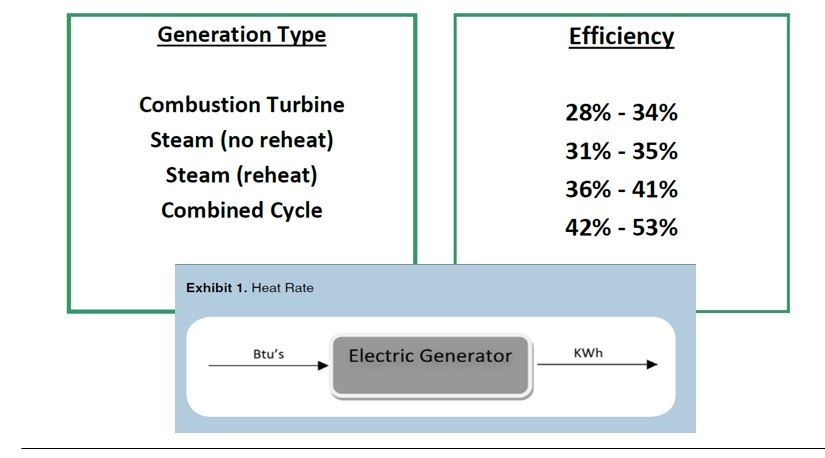# Conversions and Heat Rates

This page includes discussion and data for making thermal and other conversions.  You can download an excel file that has a whole bunch of the conversions that I have collected over the years.

## Files with Conversion Factors

To compute the LCOE of thermal you need to add the variable cost. The variable cost include the fuel cost and the O&M.

Fuel cost can be compute with the heat rate as follows

• Fuel cost/MWH = HR x Fuel Cost/MMBTU
• HR = MMBTU/MWH = kBTU/kWh= 1000 x BTU/kWh
• It is normal to see BTU/kWh for Thermal
• MMBTU/MWH = Normal HR (BTU/kWh) x 1000
• LCOE = (Cap cost/kW x CCR + FOM)/(8760 x CF) + Fuel/MWH + Var O&M/MWH

I have collected a whole bunch of conversion factors that you can download.  These are in various excel files below.

In theory, the fuel charge covers the fuel cost. The fuel cost depends on the efficiency of the plant as well as the cost of fuel.• The efficiency of a plant is defined as the input of the plant that can be measured in BTU, kCal, kJ or kWh.
• The output of the plant is measured in kWh or MWh.

## Conversion from BTU to kJ

I have trouble remembering which way the 1.05 goes when using kJ instead of BTU.  The heat rate is a little lower with kJ because:

• Conversion of input to output can be BTU/kWh, kJ/kWh or kWh/kWh
• (1 BTU = 1.05 kJ, 1 BTU=kWh/3412; 1.05 kJ/BTU)
• Example, 6800 BTU produces 1 kWh –> Heat Rate is 6800 BTU/kWh
• 6800 kBTU/MWH or 6.8 MMBTU to make MWH or (6.8 MMBTU/MWH).
• Alternatively: 6800 x 1.05 = 7140 kJ/kWhTo compute the LCOE of thermal you need to add the variable cost. The variable cost include the fuel cost and the O&M.

Fuel cost can be compute with the heat rate as follows:

• Fuel cost/MWH = HR x Fuel Cost/MMBTU
• HR = MMBTU/MWH = kBTU/kWh= 1000 x BTU/kWh
• It is normal to see BTU/kWh for Thermal
• MMBTU/MWH = Normal HR (BTU/kWh) x 1000
• LCOE = (Cap cost/kW x CCR + FOM)/(8760 x CF) + Fuel/MWH + Var O&M/MWH

## Efficiency ExampleAs 1 BTU is 1/3412 kWh, 6,800/3412 kWh in/kWh out or the efficiency is 1.99 input kWh for 1 output kWh. The efficiency is 1/1.99 or 50.25%

• 3412 BTU/kWh or 3412 kbtu/mwh or 3.412 mmbtu/mwh
• Eg. NGCC heat rate is 6,400 btu/kwh. This is input/output.
• This is 6.4 mmbtu/mwh
• If you want efficiency you need output/input or .1625 mWh/BTU
• To get efficiency you can multiply .1625 x 3.412 to get MWH out/MWH in
• This means that the efficiency is 3.412/heat rate = 3.412/6.4 = 53.3%